## Two titanium spheres approach each other head-on with the same speed and collide elastically. After the collision, one of the spheres, whose

Question

Two titanium spheres approach each other head-on with the same speed and collide elastically. After the collision, one of the spheres, whose mass is 300 g, remains at rest. (a) What is the mass of the other sphere? (b) What is the speed of the two-sphere center of mass if the initial speed of each sphere is 2.00 m/s?

in progress 0
6 months 2021-08-03T01:08:58+00:00 1 Answers 19 views 0

a)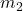= 100g

b)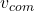= 1m/s

Explanation:

mass of one sphere ‘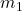‘= 300g

First final velocity of sphere= 0m/s

a) Applying the law of conservation of momentum, assuming after two spheres collide one sphere is in positive direction and the second one is in negative.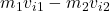=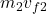As both sphere has same initial speed i,e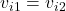Therefore,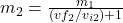—–>eq(1)

Applying conservation of kinetic energy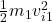+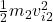=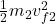So,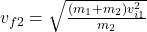=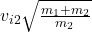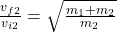By substituting the above in eq(1), we have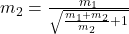Solving for, we have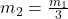=> 300/3= 100g

b) In conservation of momentum, speed of center of mass before collision equals after collision. As in above part, we assumed the directions that one sphere is in positive direction and the second one is in negative.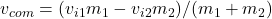=[(2×300) – (2 x 100)]/ (300+100)= 1m/s

the speed of the two-sphere center of mass is 1m/s